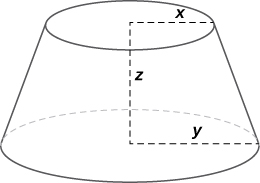$$\newcommand{\id}{\mathrm{id}}$$ $$\newcommand{\Span}{\mathrm{span}}$$ $$\newcommand{\kernel}{\mathrm{null}\,}$$ $$\newcommand{\range}{\mathrm{range}\,}$$ $$\newcommand{\RealPart}{\mathrm{Re}}$$ $$\newcommand{\ImaginaryPart}{\mathrm{Im}}$$ $$\newcommand{\Argument}{\mathrm{Arg}}$$ $$\newcommand{\norm}{\| #1 \|}$$ $$\newcommand{\inner}{\langle #1, #2 \rangle}$$ $$\newcommand{\Span}{\mathrm{span}}$$

# 3.11: The Chain Rule for Functions of Multiple Variables (Exercises)

$$\newcommand{\vecs}{\overset { \rightharpoonup} {\mathbf{#1}} }$$

$$\newcommand{\vecd}{\overset{-\!-\!\rightharpoonup}{\vphantom{a}\smash {#1}}}$$

## 13.5: The Chain Rule

In exercises 1 - 6, use the information provided to solve the problem.

1) Let $$w(x,y,z)=xy\cos z,$$ where $$x=t,y=t^2,$$ and $$z=\arcsin t.$$ Find $$\dfrac{dw}{dt}$$.

$$\dfrac{dw}{dt}=y\cos z+x\cos z(2t)−\dfrac{xy\sin z}{\sqrt{1−t^2}}$$

2) Let $$w(t,v)=e^{tv}$$ where $$t=r+s$$ and $$v=rs$$. Find $$\dfrac{∂w}{∂r}$$ and $$\dfrac{∂w}{∂s}$$.

3) If $$w=5x^2+2y^2, \quad x=−3u+v,$$ and $$y=u−4v,$$ find $$\dfrac{∂w}{∂u}$$ and $$\dfrac{∂w}{∂v}$$.

$$\dfrac{∂w}{∂u}=−30x+4y \quad\ = \quad -30(-3u + v) + 4(u - 4v) \quad = \quad 90u -30v + 4u - 16v \quad = \quad 94u - 46v$$,
$$\dfrac{∂w}{∂v}=10x−16y \quad\ = \quad 10(-3u + v) - 16(u - 4v) \quad = \quad -30u +10v - 16u + 64v \quad = \quad -46u + 74v$$

4) If $$w=xy^2,x=5\cos(2t),$$ and $$y=5\sin(2t)$$, find $$\dfrac{∂w}{∂t}$$.

5) If $$f(x,y)=xy,x=r\cos θ,$$ and $$y=r\sin θ$$, find $$\dfrac{∂f}{∂r}$$ and express the answer in terms of $$r$$ and $$θ$$.

$$\dfrac{∂f}{∂r}=r\sin(2θ)$$

6) Suppose $$f(x,y)=x+y,u=e^x\sin y,\quad x=t^2$$ and $$y=πt$$, where $$x=r\cos θ$$ and $$y=r\sin θ$$. Find $$\dfrac{∂f}{∂θ}$$.

In exercises 7 - 12, find $$\dfrac{dz}{dt}$$ in two ways, first using the chain rule and then by direct substitution.

7) $$z=x^2+y^2, \quad x=t,y=t^2$$

$$\dfrac{dz}{dt}=2t+4t^3$$

8) $$z=\sqrt{x^2+y^2},\quad y=t^2,x=t$$

9) $$z=xy,\quad x=1−\sqrt{t},y=1+\sqrt{t}$$

$$\dfrac{dz}{dt}=−1$$

10) $$z=\frac{x}{y},\quad x=e^t,y=2e^t$$

11) $$z=\ln(x+y), \quad x=e^t,y=e^t$$

$$\dfrac{dz}{dt}=1$$

12) $$z=x^4,\quad x=t,y=t$$

13) Let $$w(x,y,z)=x^2+y^2+z^2, \quad x=cost,y=sint,$$ and $$z=e^t$$. Express $$w$$ as a function of $$t$$ and find $$\dfrac{dw}{dt}$$ directly. Then, find $$\dfrac{dw}{dt}$$ using the chain rule.

$$\dfrac{dw}{dt}=2e^{2t}$$ in both cases

14) Let $$z=x^2y,$$ where $$x=t^2$$ and $$y=t^3$$. Find $$\dfrac{dz}{dt}$$.

15) Let $$u=e^x\sin y,$$ where $$x=t^2$$ and $$y=πt$$. Find $$\dfrac{du}{dt}$$ when $$x=\ln 2$$ and $$y=\frac{π}{4}$$.

$$\dfrac{du}{dt} = 2\sqrt{2}t+\sqrt{2}π$$

In exercises 16 - 33, find $$\dfrac{dy}{dx}$$ using partial derivatives.

16) $$\sin(6x)+\tan(8y)+5=0$$

17) $$x^3+y^2x−3=0$$

$$\dfrac{dy}{dx}=−\dfrac{3x^2+y^2}{2xy}$$

18) $$\sin(x+y)+\cos(x−y)=4$$

19) $$x^2−2xy+y^4=4$$

$$\dfrac{dy}{dx}=\dfrac{y−x}{−x+2y^3}$$

20) $$xe^y+ye^x−2x^2y=0$$

21) $$x^{2/3}+y^{2/3}=a^{2/3}$$

$$\dfrac{dy}{dx}=−\sqrt{\frac{y}{x}}$$

22) $$x\cos(xy)+y\cos x=2$$

23) $$e^{xy}+ye^y=1$$

$$\dfrac{dy}{dx}=−\dfrac{ye^{xy}}{xe^{xy}+e^y(1+y)}$$

24) $$x^2y^3+\cos y=0$$

25) Find $$\dfrac{dz}{dt}$$ using the chain rule where $$z=3x^2y^3,\,\,x=t^4,$$ and $$y=t^2$$.

$$\dfrac{dz}{dt}=42t^{13}$$

26) Let $$z=3\cos x−\sin(xy),x=\frac{1}{t},$$ and $$y=3t.$$ Find $$\dfrac{dz}{dt}$$.

27) Let $$z=e^{1−xy},\,\, x=t^{1/3},$$ and $$y=t^3$$. Find $$\dfrac{dz}{dt}$$.

$$\dfrac{dz}{dt}=−\frac{10}{3}t^{7/3}×e^{1−t^{10/3}}$$

28) Find $$\dfrac{dz}{dt}$$ by the chain rule where $$z=\cosh^2(xy),\,\,x=\frac{1}{2}t,$$ and $$y=e^t$$.

29) Let $$z=\dfrac{x}{y},\,\, x=2\cos u,$$ and $$y=3\sin v.$$ Find $$\dfrac{∂z}{∂u}$$ and $$\dfrac{∂z}{∂v}$$.

$$\dfrac{∂z}{∂u}=\dfrac{−2\sin u}{3\sin v}$$ and $$\dfrac{∂z}{∂v}=\dfrac{−2\cos u\cos v}{3\sin^2v}$$

30) Let $$z=e^{x^2y}$$, where $$x=\sqrt{uv}$$ and $$y=\frac{1}{v}$$. Find $$\dfrac{∂z}{∂u}$$ and $$\dfrac{∂z}{∂v}$$.

31) If $$z=xye^{x/y},\,\, x=r\cos θ,$$ and $$y=r\sin θ$$, find $$\dfrac{∂z}{∂r}$$ and $$\dfrac{∂z}{∂θ}$$ when $$r=2$$ and $$θ=\frac{π}{6}$$.

$$\dfrac{∂z}{∂r}=\sqrt{3}e^{\sqrt{3}}, \dfrac{∂z}{∂θ}=(2−4\sqrt{3})e^{\sqrt{3}}$$

32) Find $$\dfrac{∂w}{∂s}$$ if $$w=4x+y^2+z^3,\,\,x=e^{rs^2},\,\,y=\ln(\frac{r+s}{t}),$$ and $$z=rst^2$$.

33) If $$w=\sin(xyz),\,\,x=1−3t,\,\,y=e^{1−t},$$ and $$z=4t$$, find $$\dfrac{∂w}{∂t}$$.

$$\dfrac{∂w}{∂t}=-3yz\cos(xyz)−xze^{1−t}\cos(xyz)+4xy\cos(xyz)$$

In exercises 34 - 36, use this information: A function $$f(x,y)$$ is said to be homogeneous of degree $$n$$ if $$f(tx,ty)=t^nf(x,y)$$. For all homogeneous functions of degree $$n$$, the following equation is true: $$x\dfrac{∂f}{∂x}+y\dfrac{∂f}{∂y}=nf(x,y)$$. Show that the given function is homogeneous and verify that $$x\dfrac{∂f}{∂x}+y\dfrac{∂f}{∂y}=nf(x,y)$$.

34) $$f(x,y)=3x^2+y^2$$

35) $$f(x,y)=\sqrt{x^2+y^2}$$

$$f(tx,ty)=\sqrt{t^2x^2+t^2y^2}=t^1f(x,y), \quad \dfrac{∂f}{∂y}=x\frac{1}{2}(x^2+y^2)^{−1/2}×2x+y\frac{1}{2}(x^2+y^2)^{−1/2}×2y=1f(x,y)$$

36) $$f(x,y)=x^2y−2y^3$$

37) The volume of a right circular cylinder is given by $$V(x,y)=πx^2y,$$ where $$x$$ is the radius of the cylinder and $$y$$ is the cylinder height. Suppose $$x$$ and $$y$$ are functions of $$t$$ given by $$x=\frac{1}{2}t$$ and $$y=\frac{1}{3}t$$ so that $$x$$ and $$y$$ are both increasing with time. How fast is the volume increasing when $$x=2$$ and $$y=5$$? Assume time is measured in seconds.

$$\dfrac{dV}{dt} = \frac{34π}{3}\,\text{units}^3/\text{s}$$

38) The pressure $$P$$ of a gas is related to the volume and temperature by the formula $$PV=kT$$, where temperature is expressed in kelvins. Express the pressure of the gas as a function of both $$V$$ and $$T$$. Find $$\dfrac{dP}{dt}$$ when $$k=1, \dfrac{dV}{dt}=2$$ cm3/min, $$\dfrac{dT}{dt}=12$$ K/min, $$V=20 cm^3$$, and $$T=20°F$$.

39) The radius of a right circular cone is increasing at $$3$$ cm/min whereas the height of the cone is decreasing at $$2$$ cm/min. Find the rate of change of the volume of the cone when the radius is $$13$$ cm and the height is $$18$$ cm.

$$\frac{dV}{dt}=\frac{1066π}{3}\,\text{cm}^3/\text{min}$$

40) The volume of a frustum of a cone is given by the formula $$V=\frac{1}{3}πz(x^2+y^2+xy),$$ where $$x$$ is the radius of the smaller circle, $$y$$ is the radius of the larger circle, and $$z$$ is the height of the frustum (see figure). Find the rate of change of the volume of this frustum when $$x=10$$ in., $$y=12$$ in., and $$z=18$$ in.41) A closed box is in the shape of a rectangular solid with dimensions $$x,y,$$ and $$z$$. (Dimensions are in inches.) Suppose each dimension is changing at the rate of $$0.5$$ in./min. Find the rate of change of the total surface area of the box when $$x=2$$ in., $$y=3$$ in., and $$z=1$$ in.

$$\frac{dA}{dt}=12\, \text{in.}^2/\text{min}$$

42) The total resistance in a circuit that has three individual resistances represented by $$x,y,$$ and $$z$$ is given by the formula $$R(x,y,z)=\dfrac{xyz}{yz+xz+xy}$$. Suppose at a given time the $$x$$ resistance is $$100\,Ω$$, the $$y$$ resistance is $$200\,Ω,$$ and the $$z$$ resistance is $$300\,Ω.$$ Also, suppose the $$x$$ resistance is changing at a rate of $$2\,Ω/\text{min},$$ the $$y$$ resistance is changing at the rate of $$1\,Ω/\text{min}$$, and the $$z$$ resistance has no change. Find the rate of change of the total resistance in this circuit at this time.

43) The temperature $$T$$ at a point $$(x,y)$$ is $$T(x,y)$$ and is measured using the Celsius scale. A fly crawls so that its position after $$t$$ seconds is given by $$x=\sqrt{1+t}$$ and $$y=2+\frac{1}{3}t$$, where $$x$$ and $$y$$ are measured in centimeters. The temperature function satisfies $$T_x(2,3)=4$$ and $$T_y(2,3)=3$$. How fast is the temperature increasing on the fly’s path after $$3$$ sec?

$$2$$°C/sec

44) The $$x$$ and $$y$$ components of a fluid moving in two dimensions are given by the following functions: $$u(x,y)=2y$$ and $$v(x,y)=−2x$$ with $$x≥0$$ and $$y≥0$$. The speed of the fluid at the point $$(x,y)$$ is $$s(x,y)=\sqrt{u(x,y)^2+v(x,y)^2}$$. Find $$\dfrac{∂s}{∂x}$$ and $$\dfrac{∂s}{∂y}$$ using the chain rule.

45) Let $$u=u(x,y,z),$$ where $$x=x(w,t),\, y=y(w,t),\, z=z(w,t),\, w=w(r,s)$$, and $$t=t(r,s).$$ Use a tree diagram and the chain rule to find an expression for $$\dfrac{∂u}{∂r}$$.

$$\frac{∂u}{∂r}=\frac{∂u}{∂x}(\frac{∂x}{∂w}\frac{∂w}{∂r}+\frac{∂x}{∂t}\frac{∂t}{∂r})+\frac{∂u}{∂y}(\frac{∂y}{∂w}\frac{∂w}{∂r}+\frac{∂y}{∂t}\frac{∂t}{∂r})+\frac{∂u}{∂z}(\frac{∂z}{∂w}\frac{∂w}{∂r}+\frac{∂z}{∂t}\frac{∂t}{∂r})$$